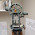## Sunday, June 29, 2014

### Bow Ties and Cylindrical Hourglasses

The Area of a Bow Tie

Consider the following bow-tie with the following dimensions:

A = length of the large (outer) line
B = length of the smaller (inner) line
H = half of the height (or length) of the bow tie

The bow tie is two trapezoids stacked on each other, with their narrow ends meeting each other.  Hence, the area of the bow tie is twice the area of a trapezoid.
The area of a trapezoid is:
A = 2 *(area of the trapezoid)
A = 2 *(1/2 * H * (A + B))
A = H * (A + B)

Fairly easy.

The Volume of a Cylindrical Hourglass

I do not think there is an official name for this, but I am naming it the cylindrical hourglass because it is built by two identical cylindrical frustums, with their smaller bases attached. Hence the volume of the hourglass is twice the volume of one of the cylindrical frustum (frustum of a cylinder).

Let:  a = A/2 = small (inner) radius,
b = B/2 = larger (outer) radius, and
h = H = height of half of the hourglass

We know the volume of a frustum of a cylinder is:
V = h/3 * π * (a^2 + a*b + b^2)
** For a derivation of the volume of frustum of a cylinder, see the next section.

Since the volume of the cylindrical hourglass is twice this volume, the final volume is:
V = 2 * h/3 * π * (a^2 + a*b + b^2)

Volume of a Frustum of a Cylinder

Imagine the cross section of the frustum placed on a Cartesian plane.  Let the bottom of the base “rest” on the x-axis as seen on the above picture.  We will calculate the volume of the frustum using the Method of Discs.  For this calculation, let the discs revolve around the y-axis.  The general formula for volume with the discs revolving around the y-axis is:

V = π * ∫ (r(y))^2 dy

The boundaries used for this calculation are:

Top Boundary:  y = h

Bottom Boundary:  y = 0

Left boundary: x = 0

Right boundary:  This is a line.  x = y * (a – b)/h + b.   See below.

Slope = change in y/change in x = (h – 0)/(a – b) = h/(a – b)

Determining the line using point (b,0) and the right boundary:

y – 0 = h/(a – b) * (x – b)
y * (a - b)/h = x – b
y * (a - b)/h + b = x

r(y) = right boundary – left boundary
r(y) = y * (a - b)/h + b – 0
r(y) = y * (a - b)/h + b

Calculating Volume:
h
V = π * ∫  (y * (a - b)/h + b)^2 dy
0

h
V = π * ∫  (a – b)^2/h^2 * y^2 + 2*b/h * (a – b) * y + b^2 dy
0
h
V = π * [ ((a – b)^2 * y^3)/(3 * h^2) + (b * y^2 * (a – b))/h + b^2 * y ]
0

V = π * h/3 * ((a – b)^2  + 3 * b * (a – b) + 3 * b^2)

V = h/3 * π * (a^2 + a*b + b^2)

--------------------------------------------------------------------------------------------------------------------------

That concludes this blog entry.  The next blog entry I plan to do is calculating the volume of a parabolic hourglass.   Thanks to all my followers and for the comments - appreciate as always.

Until next time,

Eddie

This blog is property of Edward Shore.  2014

#### 1 comment:

1.Great topic and clear explanations Eddie! Integrating these types of fomulae opens up great opportunities. The HP 35s is a great tool for exploring integration on a budget. One can get a lot of mileage from this post. Thanks for sharing!

### Retro Review: Radio Shack EC-2024

Retro Review:  Radio Shack EC-2024 Quick Facts Company:  Radio Shack Years in Production: Around 1994 Original Price: \$24.99 Bat...